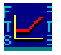Restart to return homeWhat is Gamma?

Gamma provides you with information on how delta changes. That is, it measures the sensitivity of the option’s delta with respect to changes in the underlying price.

If you have worked through the delta lesson then you already know that delta is a number that gives you important hedging information.  In particular, an option's delta tells you how many units of the underlying asset you would hold if you want to hedge the risk that the option price changes when the underlying asset price changes.

In the delta lesson you also learnt that the delta number changes as the underlying asset price changes.  This means that the number of shares required to hedge an option changes with changes in the underlying asset price.  This is often referred to as a dynamic, as opposed to a static, hedge.

Gamma, therefore, provides traders with important information regarding the rate of change of delta.  The larger an option's gamma, the more a hedge is predicted to change given a one unit (e.g. \$1) change in the underlying asset price.

Gamma Example

Suppose the gamma of an at-the-money IBM call option is 0.03 and delta is 0.46.  Suppose IBM stock price increases \$1 then IBM's delta is predicted to increase to 0.46 + 0.03 = 0.49.  You can check this for a current IBM option using the calculator.  For the current example, the delta actually changes to 0.489 which reflects the fact that the "Greeks" (delta, gamma, vega etc.) provide linear approximations.

Gamma, is also important information for designing a dynamic hedge that minimizes the required amount of adjustment needed to maintain the hedge.  A trader does this by making the hedge "gamma neutral."   This means that the position gamma is constructed to equal zero.  Now with gamma equal to zero the position's delta is less sensitive to changes in the underlying asset price and, therefore, the predicted future adjustments to the hedge will be reduced.

Graphical Insights into Gamma

We will use the Option Calculator’s Sensitivity function to gain graphical insight into the behavior of gamma.

Click on the button labelled Sensitivity and plot Gamma against the underlying Asset Price.

The red graph represents how gamma changes for different values of the underlying.  In particular, you can observe that around at-the-money is the most gamma sensitive region.  Click on the peak to see that it is just past "at-the-money" for a currently at-the-money call option.

This is useful information because, as discussed above, traders can attempt to reduce the frequency with which they have to adjust their delta hedge by making their position gamma neutral (i.e. gamma equal to 0).  The larger an option contract's gamma, the fewer option contracts are required to neutralize gamma exposure.  Fewer option contracts imply lower transaction costs.

Now plot gamma against maturity.

You will see that gamma declines as maturity increases.  This means that, all other things being equal, traders prefer short maturity options if they are trying to make a position gamma neutral.  Again, this is because fewer option contracts are need to be purchased the larger the gamma number is.

You can explore with other variations of gamma to identify factors that affect gamma.

OS Financial Trading System, PO Box 11356, Pittsburgh, PA 15238 USA, Phone 1-800-967-9897, Fax 1-412-967-5958, Email fts@ftsweb.com,